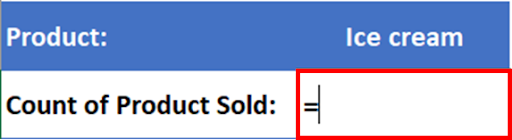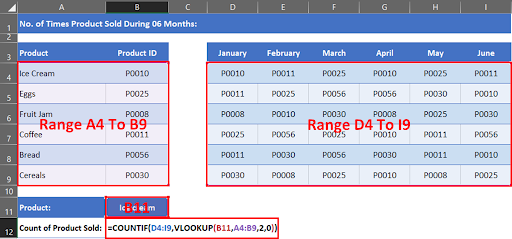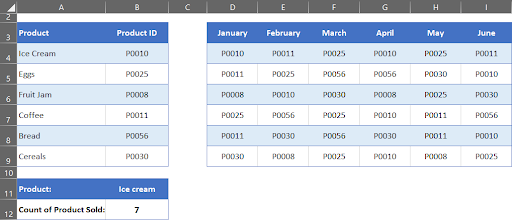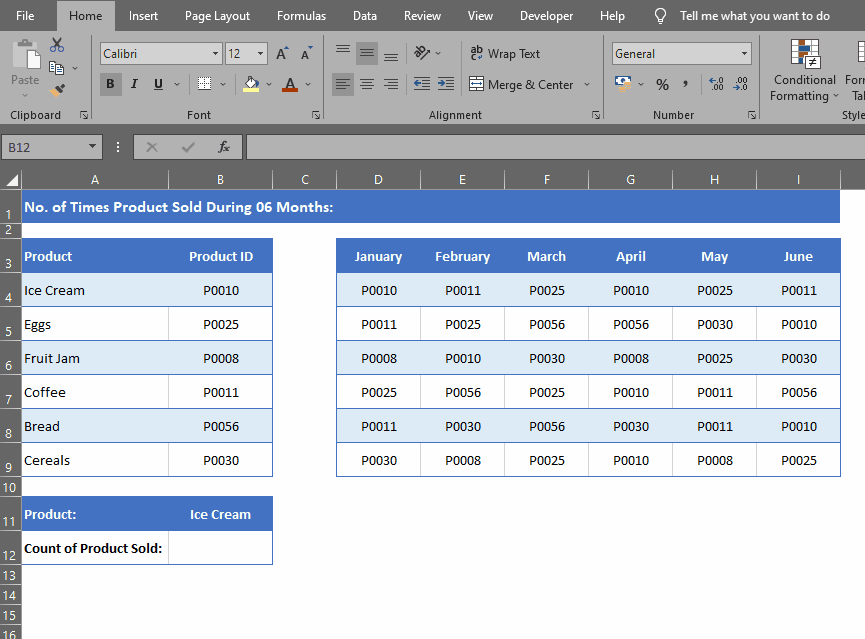# How To Use Vlookup With Countif Function In MS Excel

In this tutorial, we’ll take a look at an example to learn how to use these two functions together. Suppose we have a dataset of a grocery store. We want to find out the count of Product sold or number of times a product sold between the first 06 months in a year.

## Formula DetailsAmong MS Excel’s most versatile and powerful functions, VLOOKUP and COUNTIF are the most commonly used. The VLOOKUP function locates specific data within a table or range, while the COUNTIF function counts the number of occurrences of that data within another table or range. These two functions can be used together to search and count any value with conditions from any range.

### Step 1 – Select The Cell– Select the cell where you want to type the formula.

### Step 2 – Place Equals To Sign– Start your formula with equals to sign.

### Step 3 – Type Formula– Type formula.

### Step 4 – Press Enter– Press enter to execute the formula.

### Step 5 – Get The Count of Products Sold– Formula will display the count of products sold during the 06 months. You can change Product in Cell B11 to see the count of other Products in the table.